# Coordinates hexagon

The regular hexagon ABCDEF is given. Point A has coordinates [1; 3], and point D has coordinates [4; 7]. Calculate the sum of the coordinates of the center of its described circle.

x =  2.5
y =  5

### Step-by-step explanation:

$x=\frac{1+4}{2}=\frac{5}{2}=2\frac{1}{2}=2.5$
$y=\frac{3+7}{2}=5$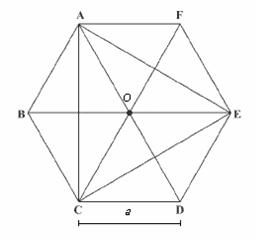Did you find an error or inaccuracy? Feel free to write us. Thank you!Tips to related online calculators
Looking for help with calculating arithmetic mean?
Line slope calculator is helpful for basic calculations in analytic geometry. The coordinates of two points in the plane calculate slope, normal and parametric line equation(s), slope, directional angle, direction vector, the length of the segment, intersections of the coordinate axes, etc.
Looking for a statistical calculator?

#### You need to know the following knowledge to solve this word math problem:

We encourage you to watch this tutorial video on this math problem:

## Related math problems and questions:

• Midpoint of segmentPoint A has coordinates [4; -11] and the midpoint of the segment AB is the point [17; -7]. What are the coordinates of point B?
• Hexagon areaThe center of the regular hexagon is 21 cm away from its side. Calculate the hexagon side and its area.
• Midpoint 6For line segment length is given: FM=8a+1, FG=42. Point M is the midpoint of FG. Find unknown a.
• Center of line segmentCalculate the distance of the point X [1,3] from the center of the line segment x = 2-6t, y = 1-4t ; t is from interval <0,1>.
• 6-gon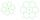Perimeter of regular hexagon is 113. Calculate its circumradius (radius of circumscribed circle).
• The coordinates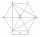The coordinates (5, 2) and (-6, 2) are vertices of a hexagon. Explain how to find the length of the segment formed by these endpoints. How long is the segment?
• 6 regular polygonIt is given 6 side regular polygon whose side is 5 cm. Calculate its content area. Compare how many more cm2 (square centimeters) has a circle in which is inscribed the 6-gon.
• Two chords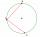There is a given circle k (center S, radius r). From point A which lies on circle k are starting two chords of length r. What angle does chords make? Draw and measure.
• Hexagon ABCDEFIn the regular hexagon ABCDEF, the diagonal AE has a length 8cm. Calculate the circumference and the hexagon area.
• Circle and hexagon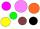Calculate the radius of a circle whose circumference is 8.4 cm longer than the inscribed regular hexagon's circumference.
• Coordinates of a centroindLet’s A = [3, 2, 0], B = [1, -2, 4] and C = [1, 1, 1] be 3 points in space. Calculate the coordinates of the centroid of △ABC (the intersection of the medians).
• Hexagon 5The distance of parallel sides of regular hexagonal is 61 cm. Calculate the length of the radius of the circle described to this hexagon.
• Chord BC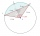A circle k has the center at the point S = [0; 0]. Point A = [40; 30] lies on the circle k. How long is the chord BC if the center P of this chord has the coordinates: [- 14; 0]?
• Center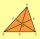In the triangle ABC is point D[1,-2,6], which is the center of the |BC| and point G[8,1,-3], which is the center of gravity of the triangle. Find the coordinates of the vertex A[x,y,z].
• Coordinates of midpointIf the midpoint of the segment is (6,3) and the other end is (8,4) what are the coordinate of the other end?
• Hexa pyramidThe base of the regular pyramid is a hexagon, which can be described by a circle with a radius of 1 m. Find the volume of the pyramid 2.5 m high.
• Perpendicular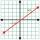What is the slope of the perpendicular bisector of line segment AB if A[9,9] and B[9,-2]?